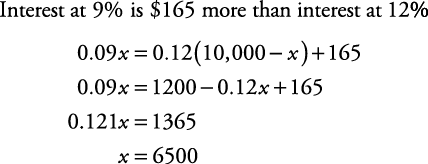## Simple Interest

The formula necessary to solve simple interest problems is

I = PRT##### Example 1

Jim has \$10,000 to invest. He will invest part at 9% annual interest and the remaining part at 12% annual interest. After one year, he expects to earn \$165 more from the 9% investment than from the 12% investment. How much will he invest at each rate?

Let x = amount invested at 9%. ThenThe sentence “he expects to earn \$165 more from the 9% investment than from the 12% investment” is translated into the following.The check is left to you. Jim will invest \$6500 at 9% and \$3500 at 12%.